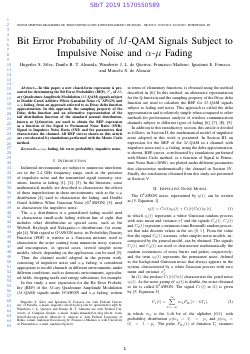##### Bit Error Probability of M-QAM Signals Subject to Impulsive Noise and $$\alpha$$-$$\mu$$ Fading

###### Abstract
In this paper, a new closed-form expression is presented for determining the Bit Error Probability (BEP), $$P_e$$, of M-ary Quadrature Amplitude Modulation (M-QAM) signals subject to Double Gated Additive White Gaussian Noise (G²AWGN) and $$\alpha$$-$$\mu$$ fading, from an approach referred to as Dirac delta function approximation. In this approach, the sampling property of the Dirac delta function and an alternative representation of the tail distribution function of the standard normal distribution, known as Q-function, are used to obtain the BEP expression as a function of the Signal to Permanent Noise Ratio (SNR), Signal to Impulsive Noise Ratio (SNI) and the parameters that characterizes the channel. All BEP curves shown in this article are corroborated by simulations performed with the Monte Carlo method.